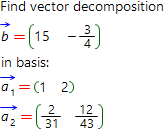# Find vector decomposition in basis, online calculatorArbitrary vector of any n-dimensional space can be expressed in the form of the linear combination of some basis vectors of this n-dimensional space. Such the decomposition is uniquely one.

Decomposition of the arbitrary n-dimensional vectorin the basis formed by linearly independent system of n-dimensional vectors1 ,2 , ... ,n , has the following form:= λ11 + λ22 + ... + λnn

, where λi − some constants called the coefficients of the decomposition (linear combination) of the vectorin basis1 ,2 , ... ,n.

Our online calculator is able to find the decomposition of vector in basis with step by step solution for free.

Decompose vector by basis1 by:2 by:by:1 = { }2 = { }= { }